## 搬砖的攻城狮

14帖子
0回复
898积分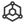ID:000040
baltamatica

1、系统的思想

2、系统的定义中国载人航天官方网站 中国载人航天工程网 http://www.cmse.gov.cn/gygc/xtzc/htyxt/。

 钱学森．论宏观建筑与与微观建筑：杭州出版社，2001

0 2022-12-13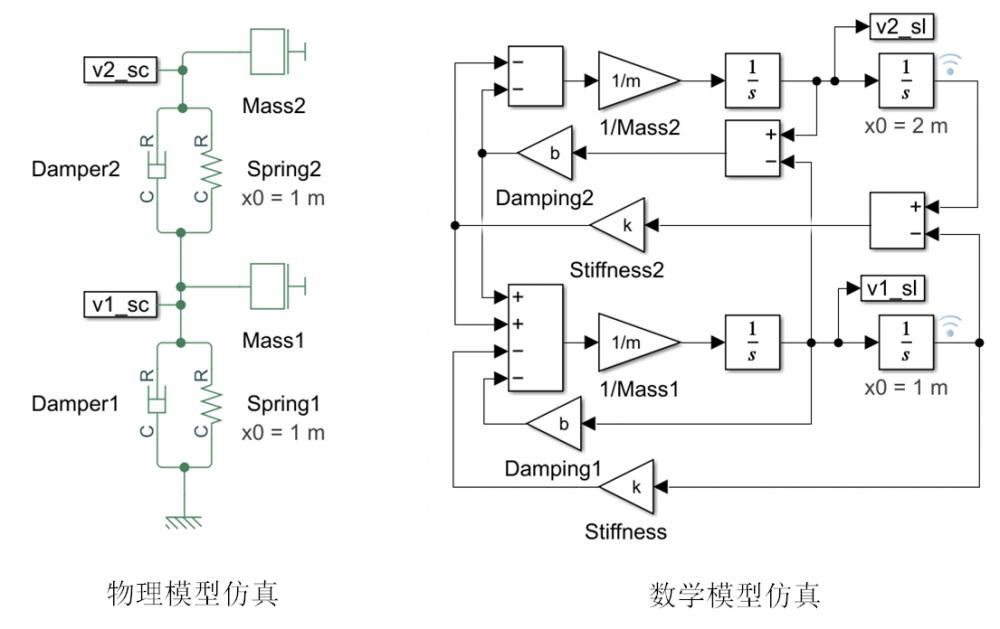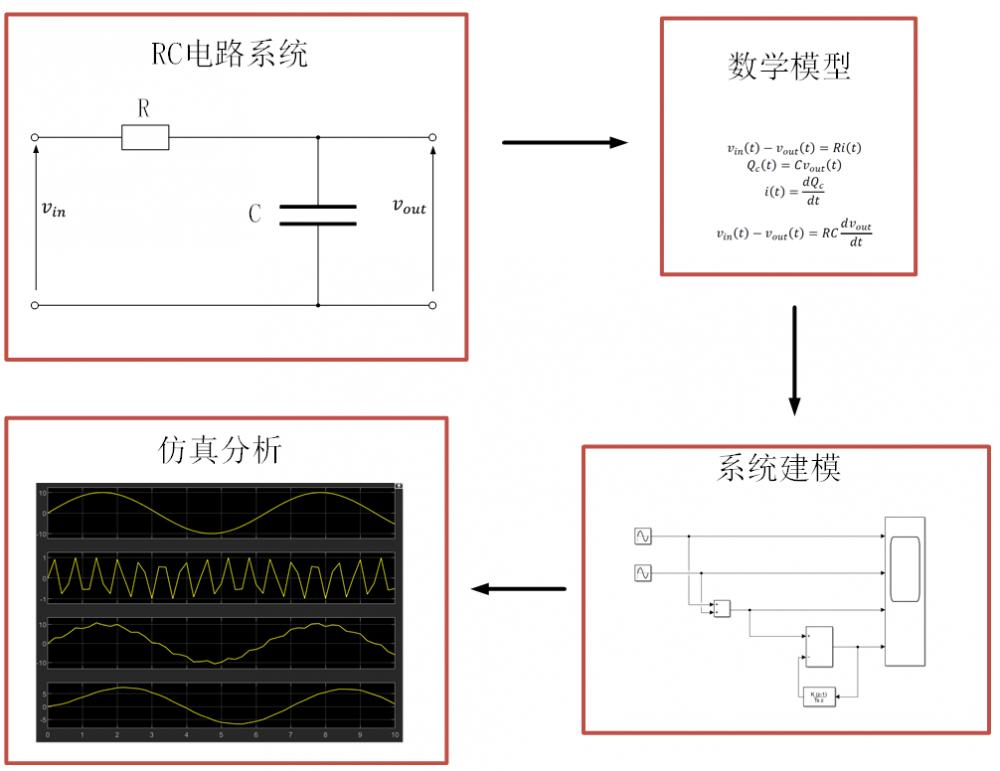福州大学《系统仿真技术》研究生课程

 http://www.bilibili.com/video/BV16E411A7zv?p=3&vd_source=f52c61024bca044c638d9c2fb92b3ede.

1 2022-12-19

LabVIEW是另一款功能强大的系统仿真软件，它可以帮助我们快速构建复杂的系统模型，并且可以模拟多种不同的系统。此外，LabVIEW还提供了丰富的可视化工具，可以帮助我们更好地理解系统的运行情况。LabVIEW主要用于开发测量或控制系统。

Modelica是一种用于建模和仿真复杂系统的面向对象的编程语言。它提供了一种灵活的方法来描述系统的行为，并且可以用于模拟和分析复杂的系统。Modelica语言支持多种类型的模型，包括动态系统、静态系统、热系统、流体系统和电气系统。它还支持多种类型的仿真，包括时间域仿真、频率域仿真和状态空间仿真。

OpenModelica属于开源模拟软件包。OpenModelica是一个功能强大的多功能工具，可用于模拟各种系统。它被设计为易于使用，并具有广泛的功能，包括一个预先构建的模型库、一种强大的编程语言和一个图形用户界面。

Scilab是一款开源的科学计算软件，它可以用于数值计算、矩阵运算、绘图、科学可视化、数据分析、模拟仿真等。它拥有丰富的函数库，可以满足各种科学计算需求。Scilab可以运行在Windows、Linux、Mac OS X等操作系统上，支持多种编程语言，如C、C++、Fortran等，可以与其他软件进行交互，如Matlab、Maple、Mathematica等。

0 2023-02-13

(1) 设拟合多项式为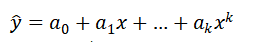(2) 各点到这条曲线的距离之和，即误差平方和为：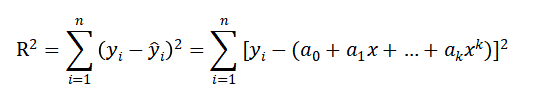(3) 根据最小二乘原理，等式右边求ai偏导数：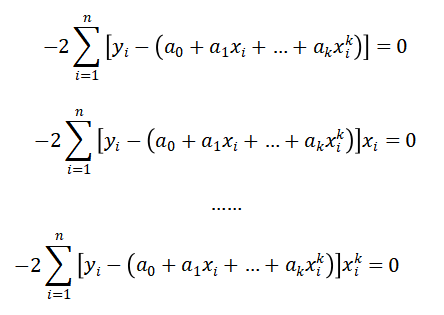(4) 化简得：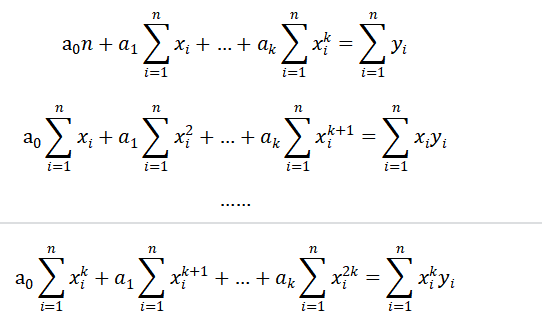(5) 化成矩阵形式：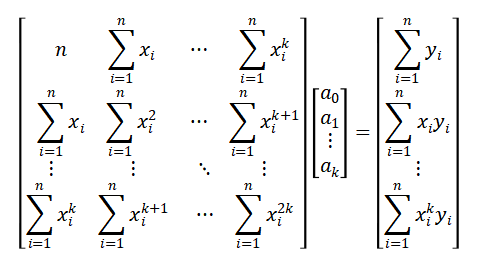(6) 化简得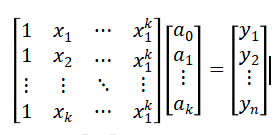(7) 求解上述方程组，即可得到系数矩阵，从而得到拟合多项式。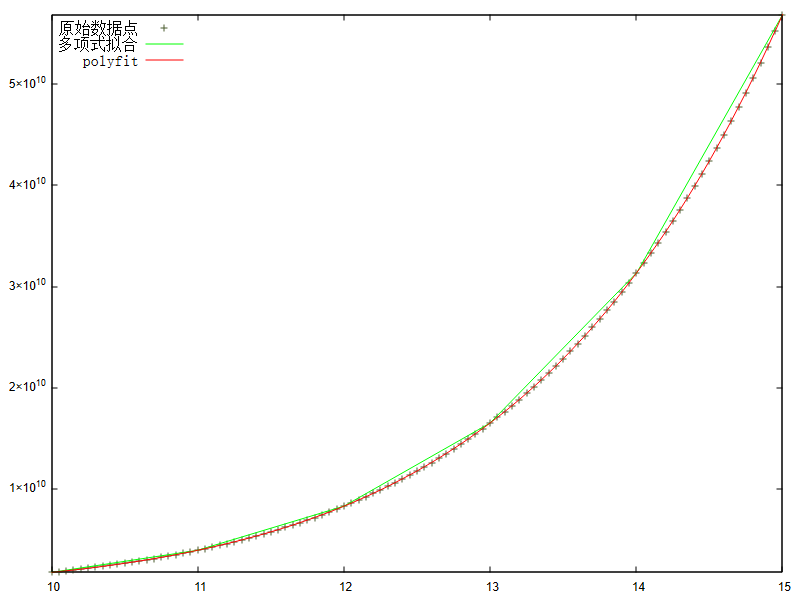附录代码：

```clear all
close all
clc
N=10;%多项式阶数
%原始数据点
X=10:0.05:15;
M=length(X);
p=[5.6,2.5,3.3,0.18,0.29,2.4,4.8,1.2,7.1,1];
Y=zeros(1,M);
for i=1:10
Y=Y+p(i)*X.^(i-1);
end
% Y=Y+unifrnd(-2e9,2e9,1,M);
%多项式拟合
X1=zeros(N+1,M);
for i=1:M
X1(1,i)=1;
end
for i=2:N+1
X1(i,:)=power(X,i-1);   %构造系数矩阵
end
XX=X1';
P_n=(XX'*XX)\XX'*Y';
X_n=min(X):max(X);
Y_n=zeros(1,length(X_n));
for i=1:N+1
Y_n=Y_n+P_n(i)*X_n.^(i-1);
end
%polyfit
P=zeros(1,N);
P=polyfit(X,Y,N);
X_polyfit=zeros(1,M);
Y_polyfit=zeros(1,M);
X_polyfit=linspace(min(X),max(X),M);
Y_polyfit=polyval(P,X_polyfit);
figure(1);
plot(X,Y,'.');
hold on
plot(X_n,Y_n,'g');
hold on
plot(X_polyfit,Y_polyfit,'r');
legend('原始数据点','多项式拟合','polyfit','Location','northwest');```

0 2023-03-01

## 1 ode45函数的原理

ode45表示采用四阶-五阶Runge-Kutta算法，它用4阶方法提供候选解，5阶方法控制误差，是一种自适应步长（变步长）的常微分方程数值解法，其整体截断误差为(Δx)^5。解决的是非刚性常微分方程。

ode45是解决数值解问题的首选方法，若长时间没结果，应该就是刚性的，可换用ode15s试试。

## 2 经典四阶Runge-Kutta介绍

y' =f(t,y)，y(t0)=y0

y(n+1)=y(n)+h/6*(k1+2*k2+2*k3+k4)

k1=f(t(n),y(n))

k2=f(t(n)+h/2,y(n)+h/2*k1)

k3=f(t(n)+h/2,y(n)+h/2*k2)

k4=f(t(n)+h,y(n)+h*k3)

k1是时间段开始时的斜率；

k2是时间段中点的斜率，通过欧拉法采用斜率k1来决定y在点tn+h/2的值；

k3也是中点的斜率，但是这次采用斜率k2决定y值；

k4是时间段终点的斜率，其y值用k3决定。

RK4法是四阶方法，也就是说每步的误差是h阶，而总积累误差为h阶。

## 3 ode45函数求解常微分方程举例

dx1 = x2  x1(0) = 1;

dx1/dt = 7(1-x1²)*x2-x1  x2(0) = 0;

function fy = vdp(t,x)

fy = [x(2);7*(1-x(1)^2)*x(2)-x(1)];

>> y0 = [1;0];

>> [t,x] = ode45(@vdp,[0 40],y0);

>> y = x(:,1);

>> plot(t,y)

>> xlabel('t')

>> ylabel('y')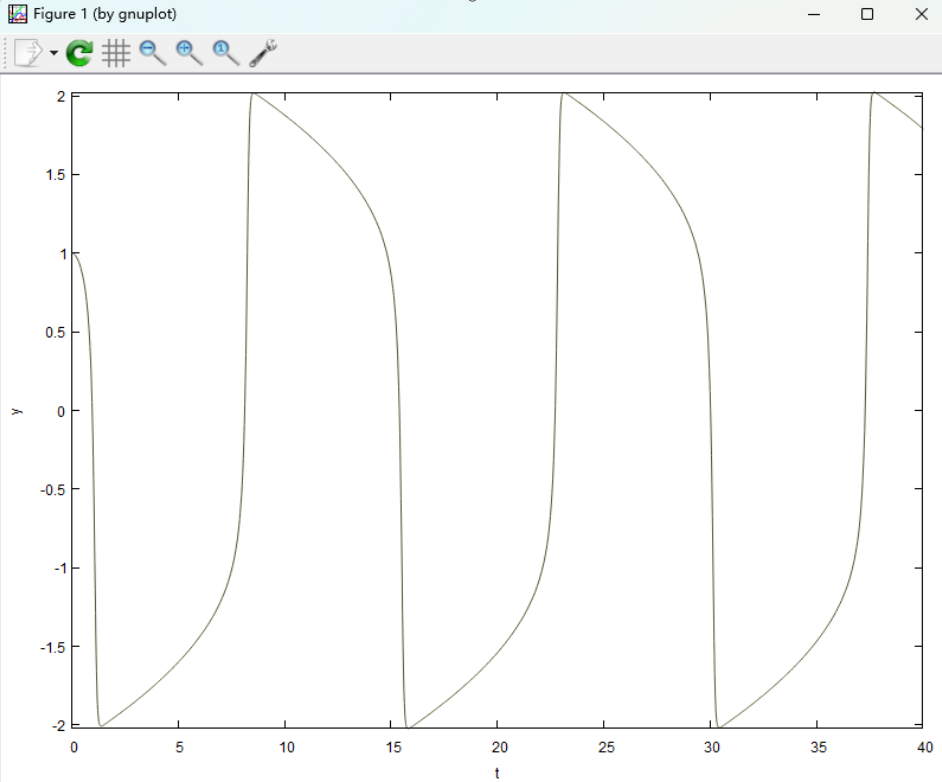0 2023-03-09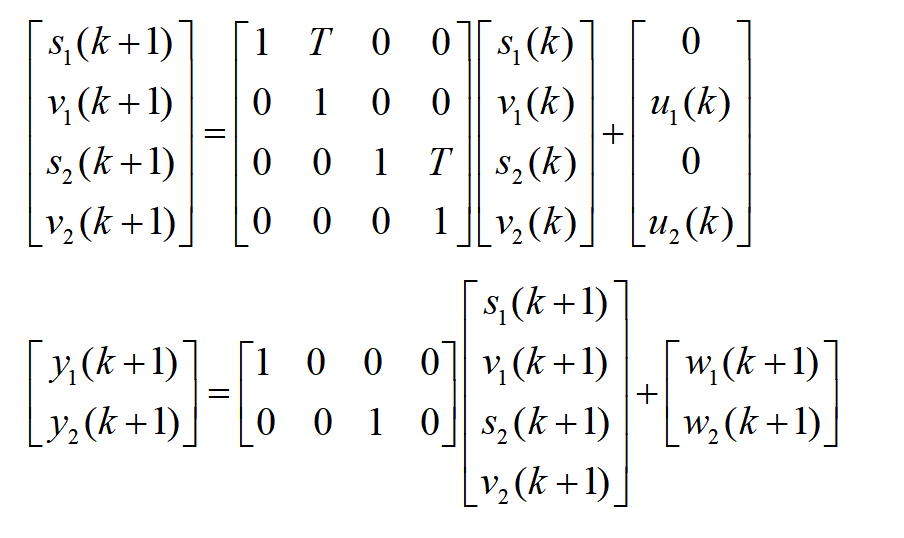s1(k)、v1(k)和y1(k)分别为径向距离、速度和观测值，而s2(k)、v2(k)和y2(k)分别为横向距离、速度和观测值。u1(k)和u2(k)是状态噪声，是目标速度的波动；w1(k)和w2(k)是观测噪声；四种噪声的均值都为0，呈高斯分布，互不相关。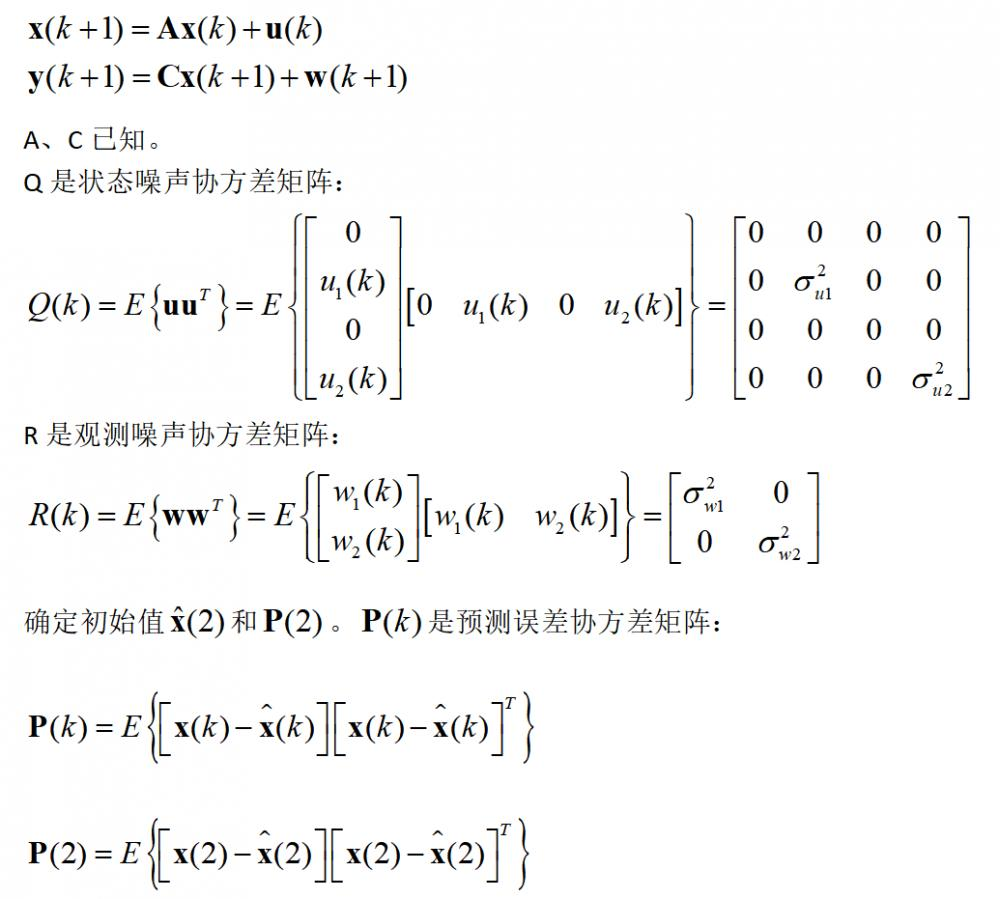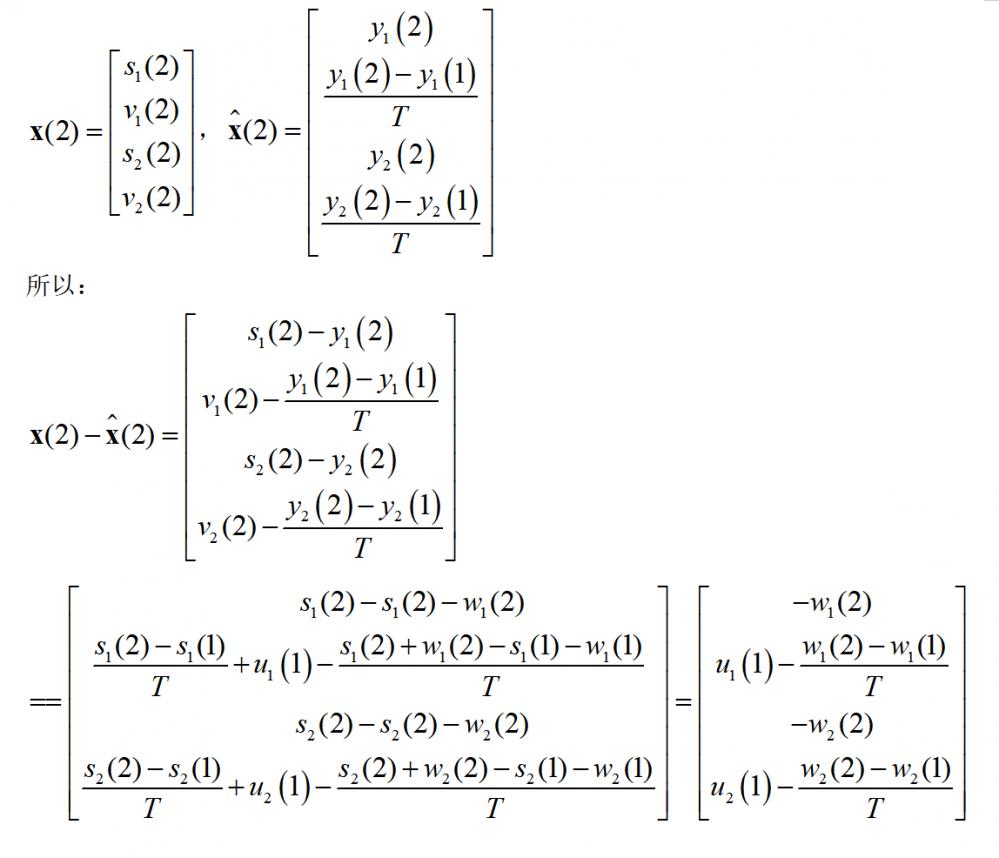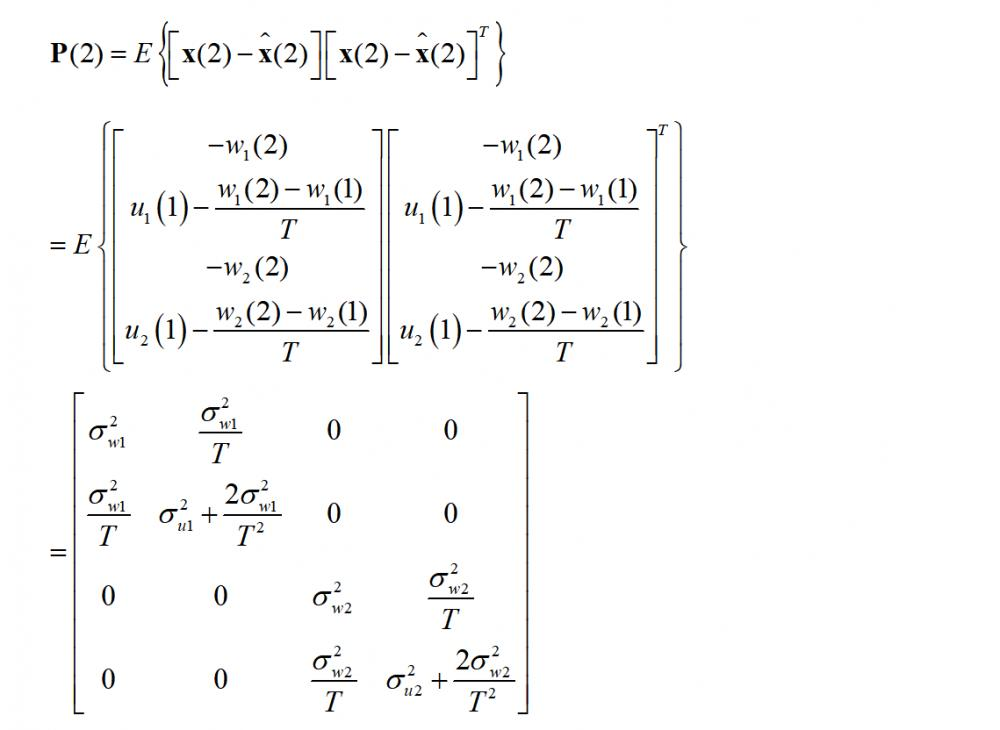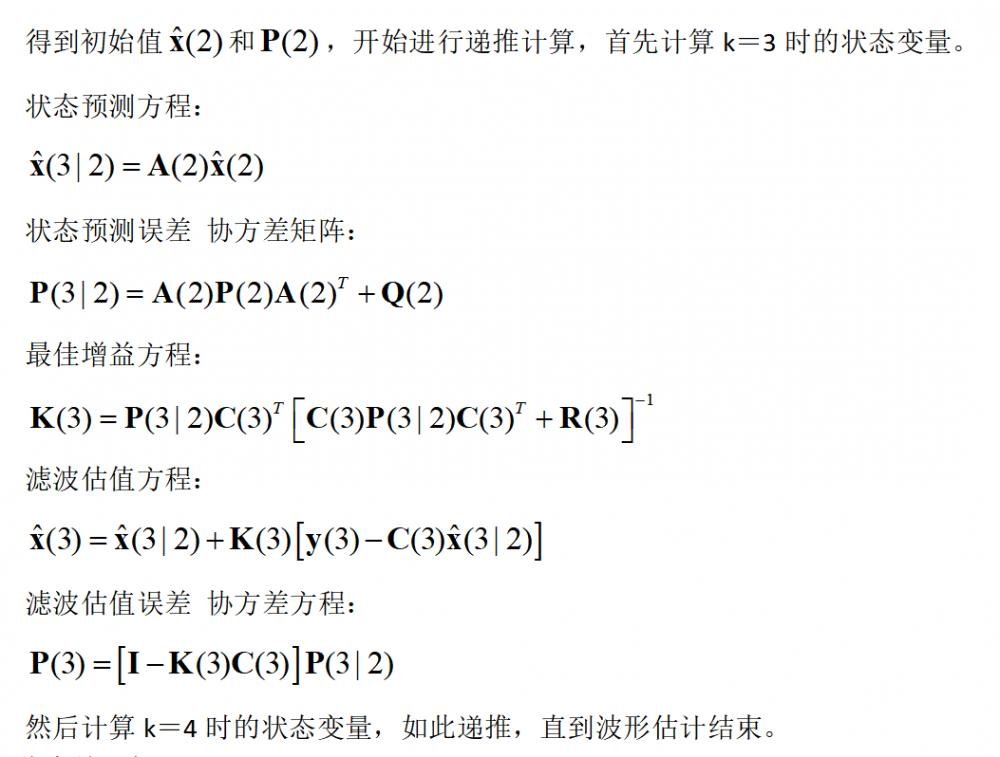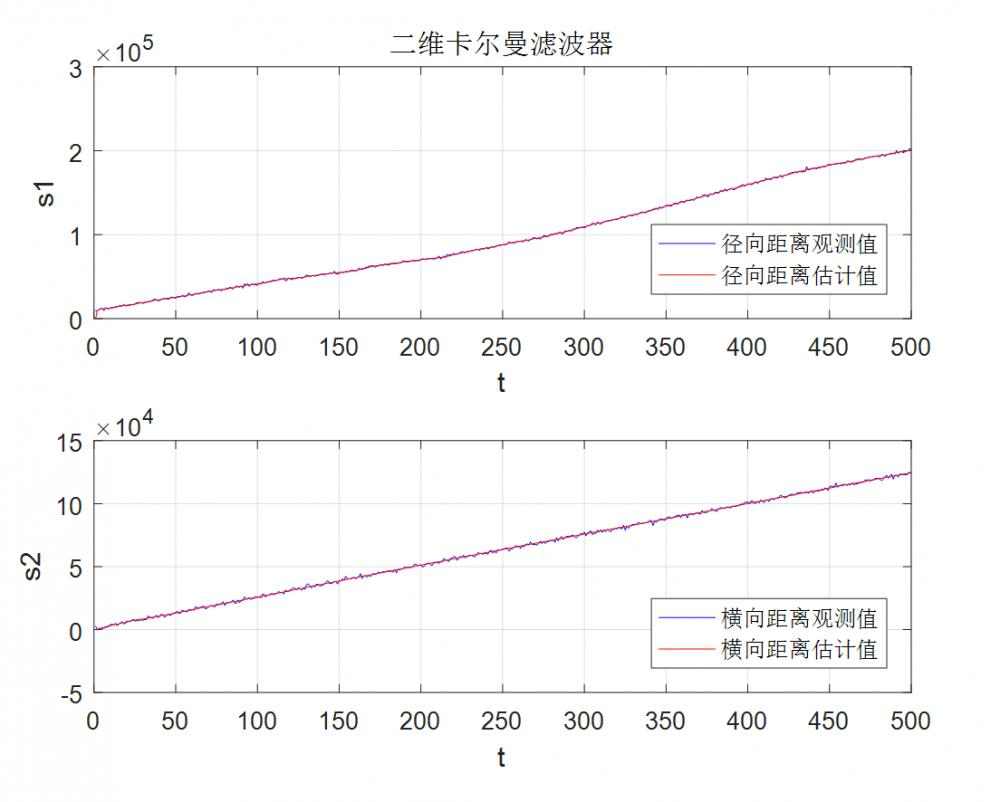`clear allclose allclcT=1;sigma_u1=300;sigma_u2=0.12;sigma_w1=900000;sigma_w2=900000;N=500;u1=sqrt(sigma_u1).*randn(1,N);u2=sqrt(sigma_u2).*randn(1,N);w1=sqrt(sigma_w1).*randn(1,N);w2=sqrt(sigma_w2).*randn(1,N);A=[     1,T,0,0;    0,1,0,0;    0,0,1,T;    0,0,0,1;    ];C=[     1,0,0,0;    0,0,1,0;    ];Q=[                       %状态噪声协方差矩阵    0,0,0,0;    0,sigma_u1,0,0;    0,0,0,0;    0,0,0,sigma_u2;    ];R=[                         %观测噪声协方差矩阵    sigma_w1,0;    0,sigma_w2;    ];I=[     1,0,0,0;    0,1,0,0;    0,0,1,0;    0,0,0,1;    ];x0=[10000;300;900;256;];%初始值% y0=[10000;0;];x=zeros(4,N);y=zeros(2,N);x(:,1)=x0;% y(:,1)=y0;x_calculation=zeros(4,N);  %状态滤波估计值u=[zeros(1,N);u1;zeros(1,N);u2];w=[w1;w2];y(:,1)=C*x(:,1)+w(:,1);P2=[    sigma_w1,  sigma_w1/T,               0,         0;    sigma_w1/T,sigma_u1+2*sigma_w1/(T^2),0,         0;    0,         0,                        sigma_w2,  sigma_w2/T;    0,         0,                        sigma_w2/T,sigma_u2+2*sigma_w2/(T^2);    ];p=P2;for i=1:N-1      x(:,i+1)=A*x(:,i)+u(:,i);%真实值    y(:,i+1)=C*x(:,i+1)+w(:,i+1);                 endx_calculation(:,2)=[y(1,1) , (y(1,2)-y(1,1))/T ,y(2,2), (y(2,2)-y(2,1))/T]';for i=3:N    x1=A*x_calculation(:,i-1);%状态预测方程    p1=A*p*A'+Q;  %状态预测误差协方差矩阵    K=p1*C'*inv(C*p1*C'+R);   %最佳增益方程    x_calculation(:,i)=x1+K*(y(:,i)-C*x1);  %滤波估值方程    p=(I-K*C)*p1; %滤波估值误差 协方差方程end t=1:N; figure(4)plot(t,y(1,:),'b',t,x_calculation(1,:),'r');xlabel('t');ylabel('s1');legend('径向距离观测值','径向距离估计值','Location','southeast');title('二维卡尔曼滤波器');figure(5)plot(t,y(2,:),'b',t,x_calculation(3,:),'r');xlabel('t');ylabel('s2');legend('横向距离观测值','横向距离估计值','Location','southeast');`

0 2023-03-09

## 1 模型假设

1) 忽略转向系的影响，以前、后轮转角作为输入；

2) 汽车只进行平行于地面的平面运动，而忽略悬架的作用；

3) 汽车前进（纵轴）速度不变，只有沿y轴的侧向速度和绕z轴的横摆运动(ay<0.4g) ；

4) 驱动力不大，对侧偏特性无影响；

5) 忽略空气阻力；

6) 忽略左右轮胎因载荷变化引起轮胎特性的变化；

7) 忽略回正力矩的变化。

## 2 模型建立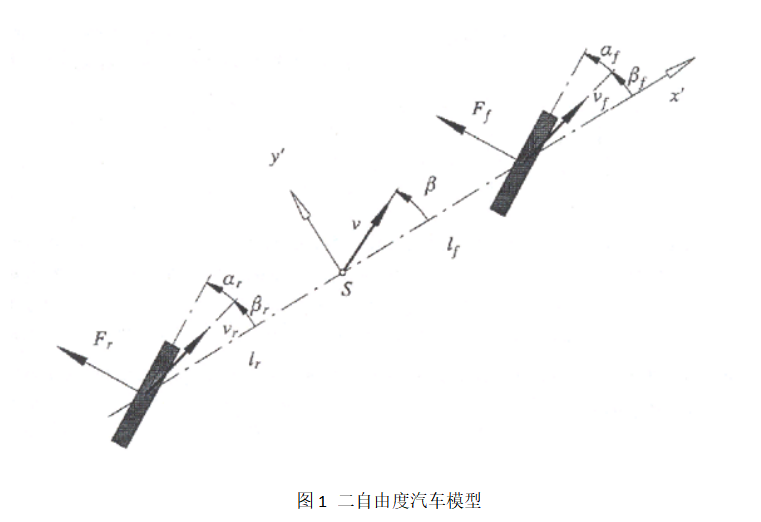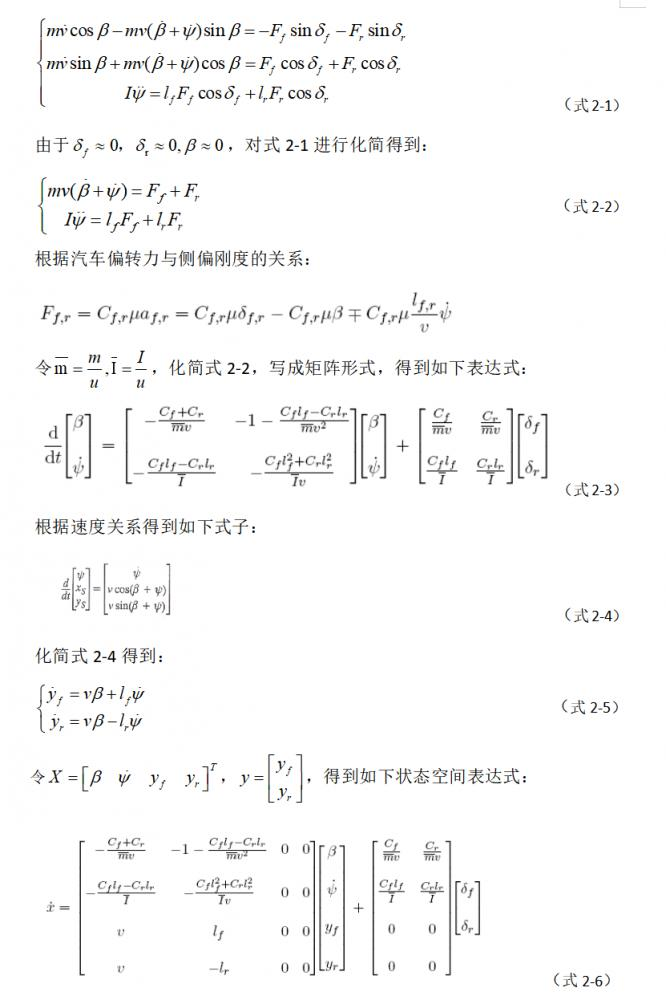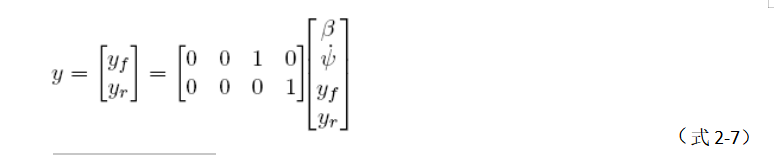## 3 模型仿真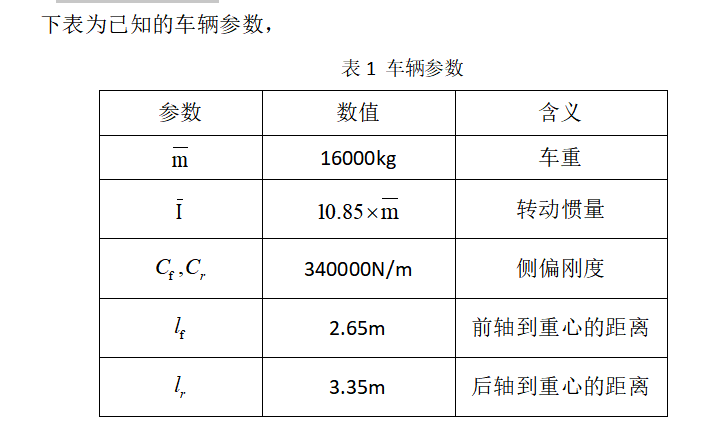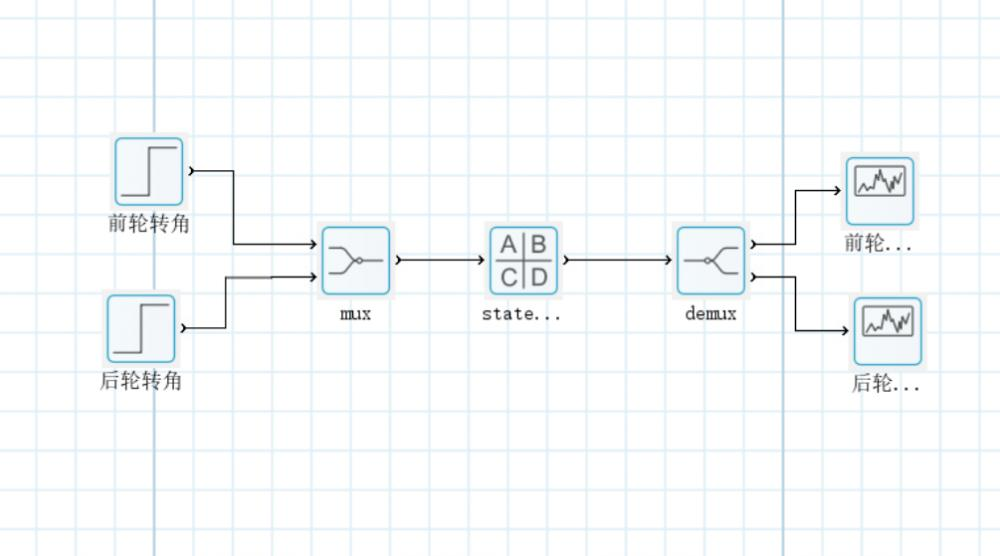（1）在前轮偏转角为1，后轮偏转角为1，车速为40km/h的情况下，输出前后轮的横向位移情况，输出结果如图3。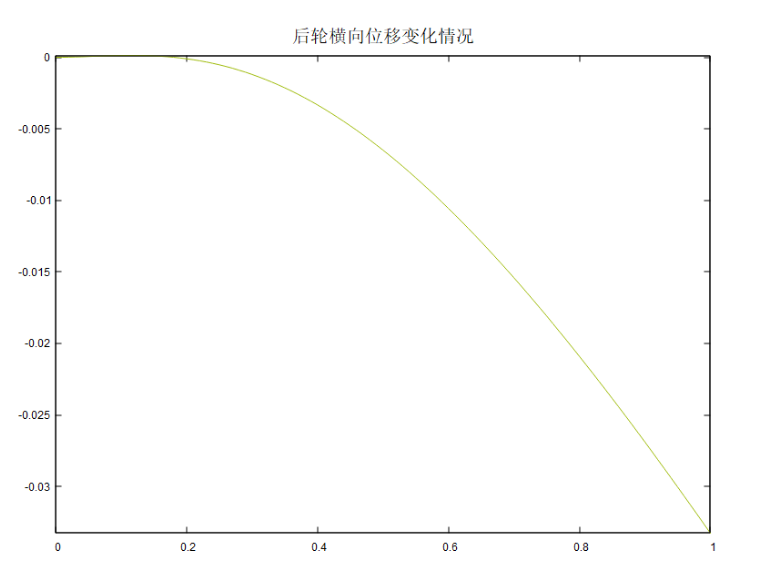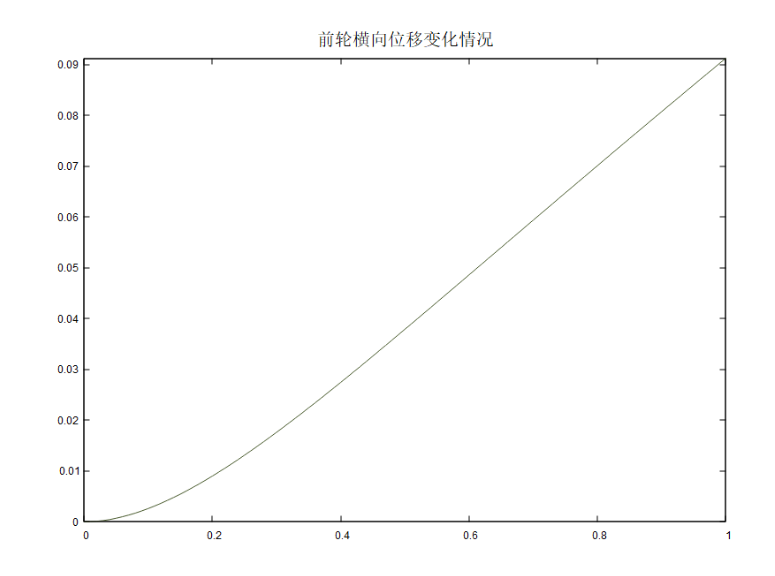## 附录

baltamatica代码如下

clc;clear;close all;

%% 基本车辆参数

v=40/3.6;%输入为km/h，方程单位为m/s

m=16000;%车重

I=10.85*m;%转动惯量

cf=340000;%侧偏刚度

cr=cf;

lf=2.65;%前轴到重心的距离

lr=3.35;%后轴到重心的距离

a=pi/180;

%% 组装矩阵

A=[-(cf+cr)/(m*v)      -1-(cf*lf-cr*lr)/(m*v^2)          0 0

-(cf*lf-cr*lr)/I     -(cf*lf^2+cr*lr^2)/(I*v)            0 0

v                          lf                0 0

v                          -lr                0 0];

B=[cf/(m*v)   cr/(m*v)

cf*lf/I     cr*lr/I

0           0

0           0];

C=[0 0 1 0

0 0 0 1];

D=zeros(2,2);

0 2023-03-21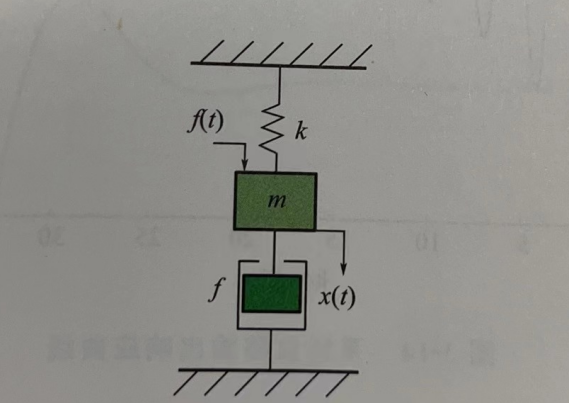m dx²/dt² + c dx/dt + kx = f(x)

dX/dt = AX(t) + BU

Y = CX(t) + DU

G(s) = 1/(s^2 + 0.3 s + 5);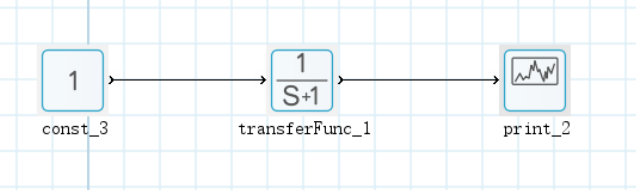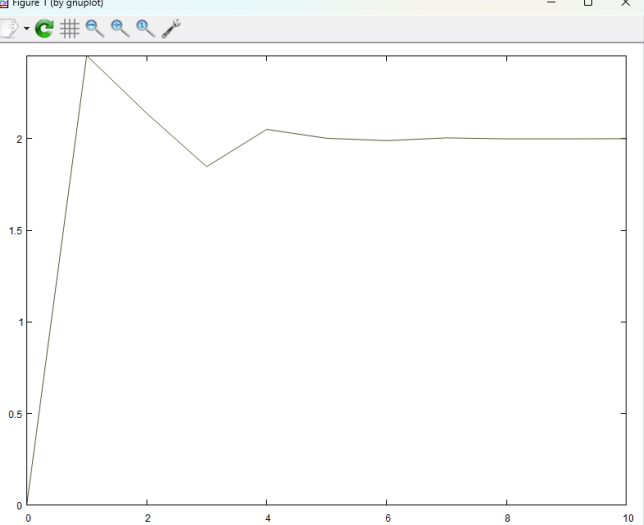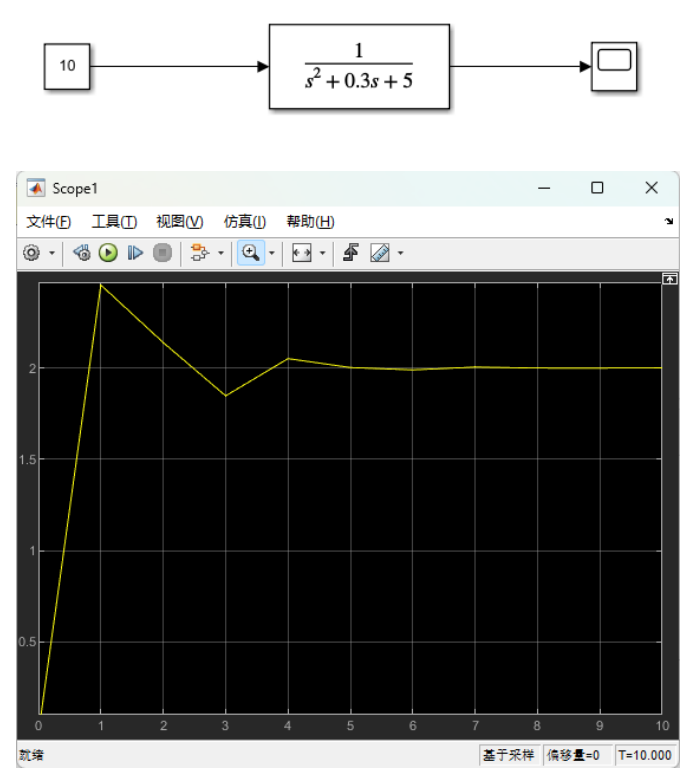0 2023-03-23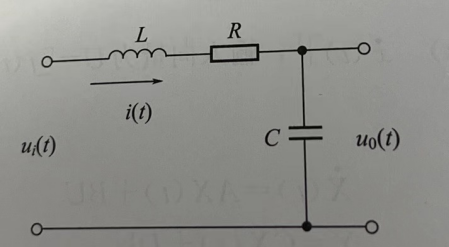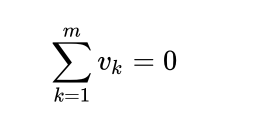L di(t)/dt + 1/C∫i(t)dt + Ri(t) = ui(t)

LC d²u0(t)/dt² + RC du0(t)/dt + u0(t) = ui(t)

d²u0(t)/dt² + 0.5 du0(t)/dt + 0.25u0(t) =  0.25ui(t)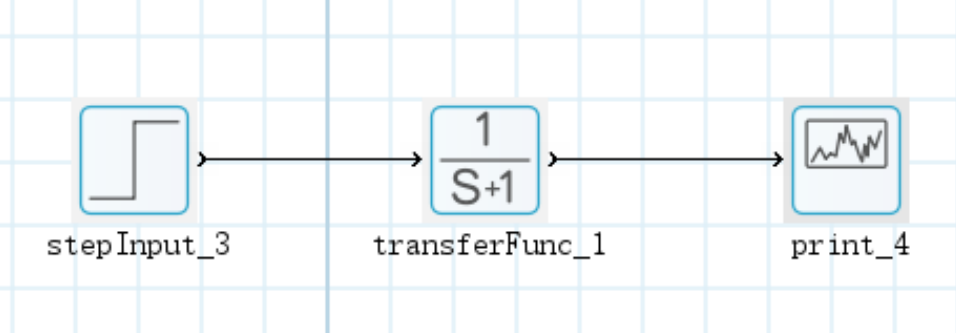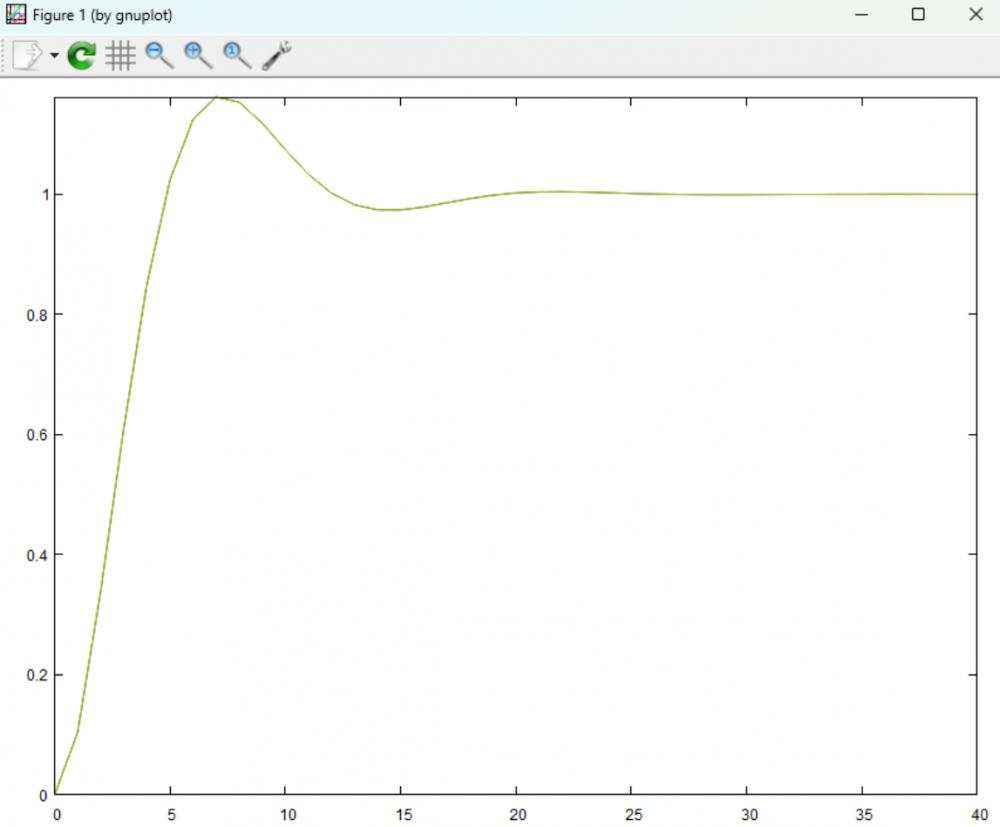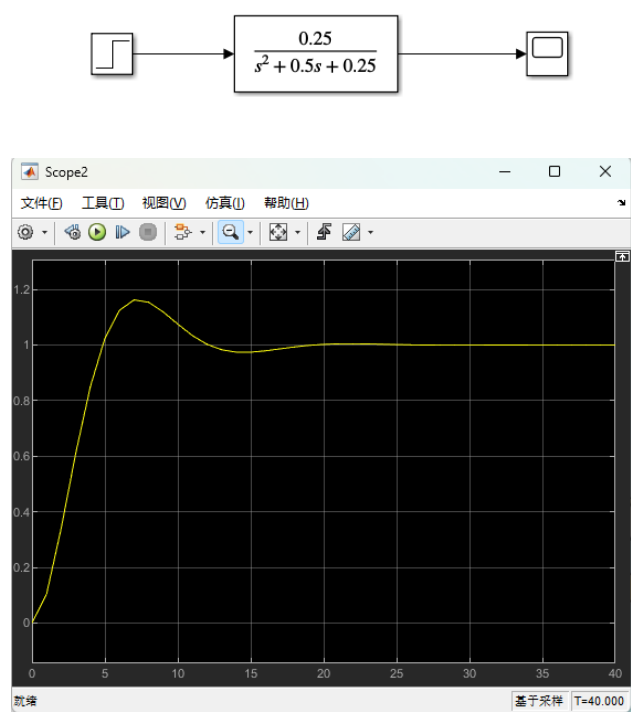0 2023-03-23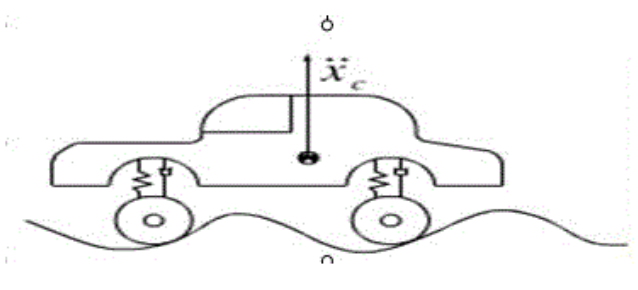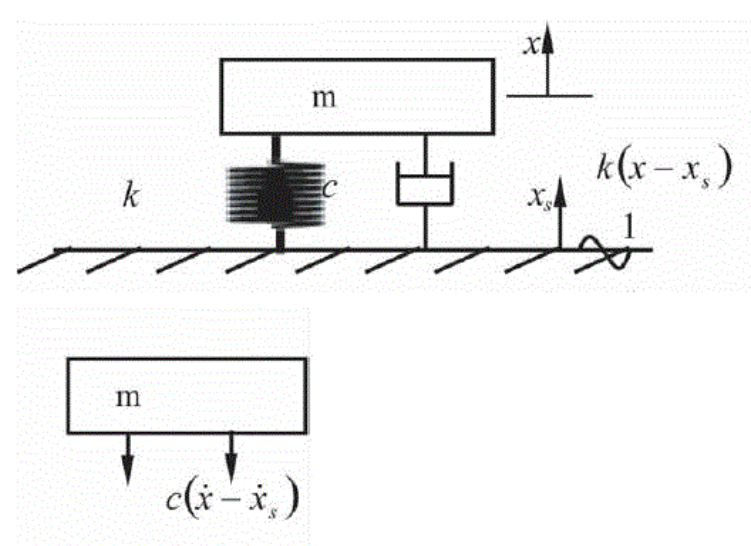Xs =asinwt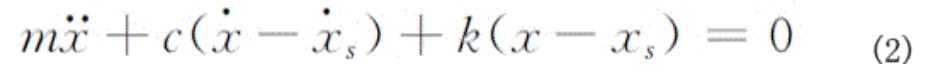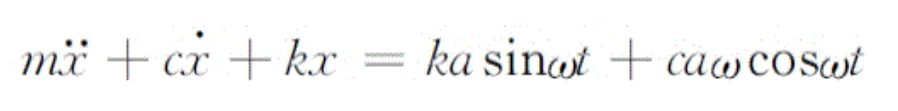Px=P0sinwt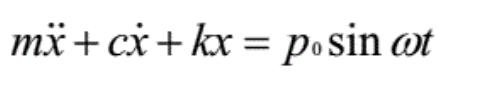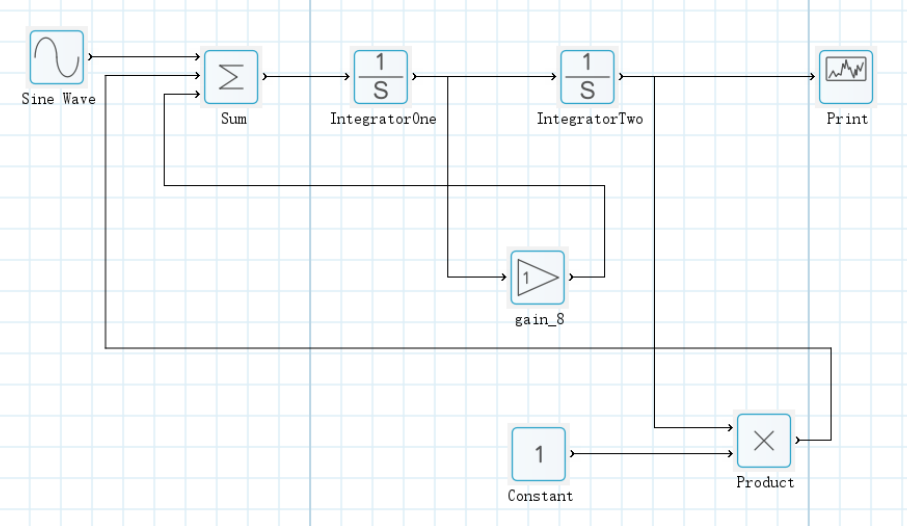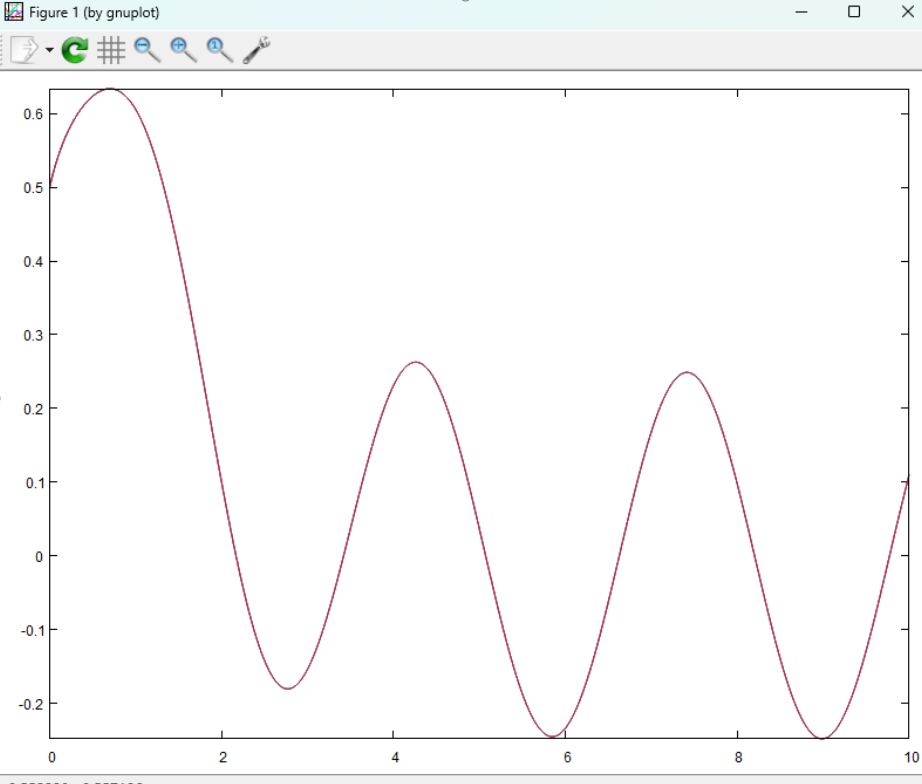0 2023-03-31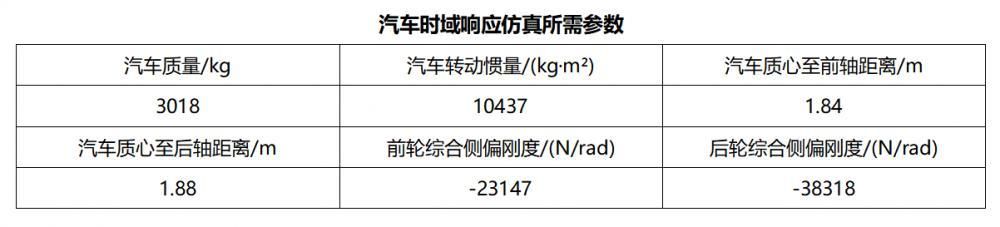G1(s) = ((s - a11)*b21 + a21*b11) / s²- (a11 + a22)*s + a11*a22 - a12*a21;

G2(s) = ((s - a22)*b11 + a12*b21) / s²- (a11 + a22)*s + a11*a22 - a12*a21;

a11 = (Ka1 + Ka2) / mu;     a12 = (aKa1 - bKa2 - mu²) / mu²;

a21 = (aKa1 - bKa2) / Iz;     a22 = (a²Ka1 + b²Ka2) / Iz*u;

b11 = -Ka1 / mu;            b21 = -aKa1/Iz；

### 汽车横摆角度速度传递函数

>> m=3018; Iz=10437; a=1.84; b=1.88; k1=-23147; k2=-38318;

>> u= [10 20 30];

>> a11 = (k1 + k2)/m./u;

>> a12 = (a*k1 - b*k2 -m.*u^2)/m./u^2;

>> a21 = (a * k1 - b * k2)/Iz;

>> a22=(a^2*k1 + b^2*k2)/Iz./u;

>> b11 = -k1/m./u;

>> b21 = -a*k1/Iz;

>> b1 = b21;

>> b2 = a21*b11-a11*b21;

>> b3 = -a11-a22;

>> b4 = a11.*a22-a12.*a21;

>> num = [b1 b2];

>> den = [1,b3,b4];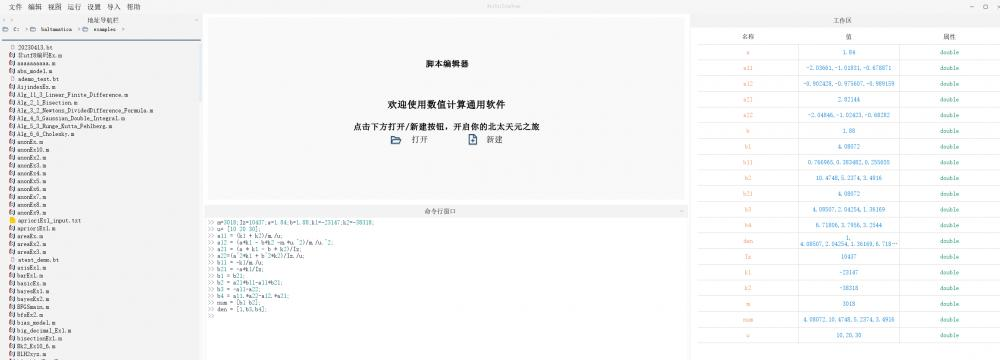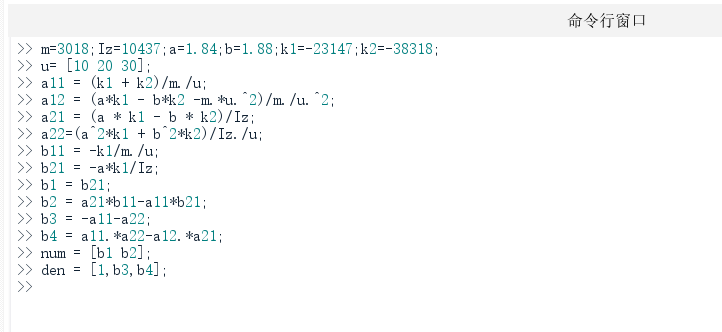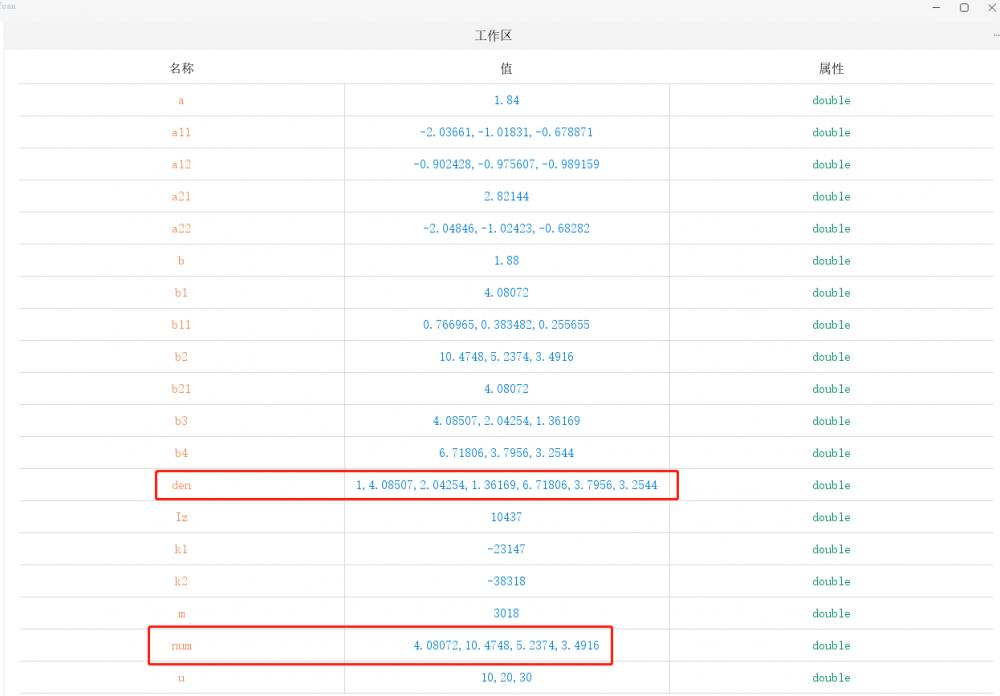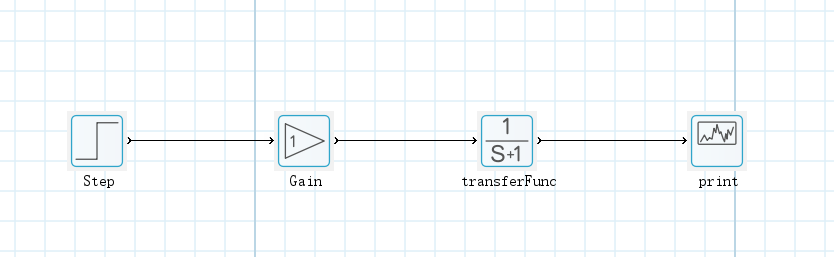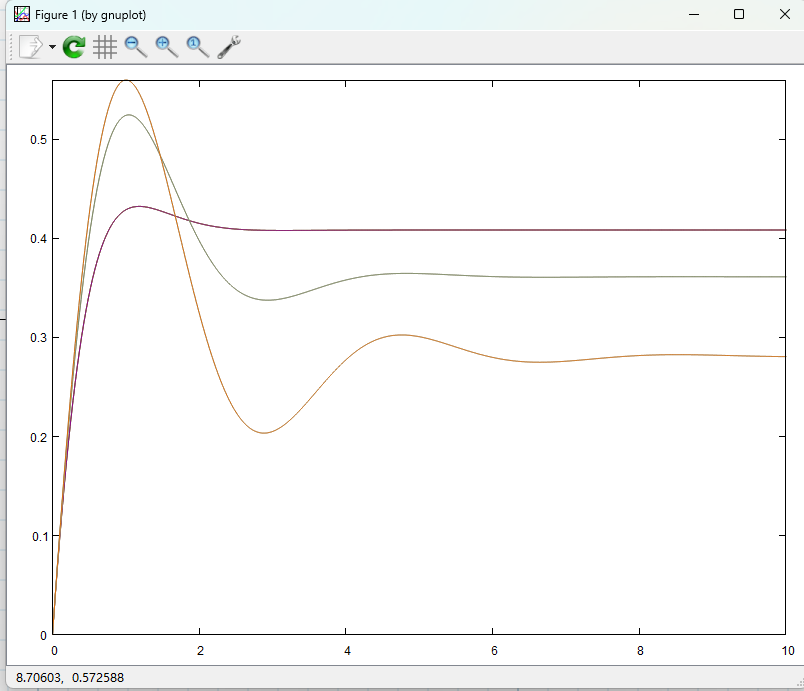### 质心偏侧角传递函数

>> m=3018;Iz=10437;a=1.84;b=1.88;k1=-23147;k2=-38318;

>> u= [10 20 30];

>> a11 = (k1 + k2)/m./u;

>> a12 = (a*k1 - b*k2 -m.*u.^2)/m./u.^2;

>> a21 = (a * k1 - b * k2)/Iz;

>> a22=(a^2*k1 + b^2*k2)/Iz./u;

>> b11 = -k1/m./u;

>> b21 = -a*k1/Iz;

>> b1 = b11;

>> b2 = a12*b21-a22*b11;

>> b3 = -a11-a22;

>> b4 = a11.*a22-a12.*a21;

>> num = [b1 b2];

>> den = [1,b3,b4];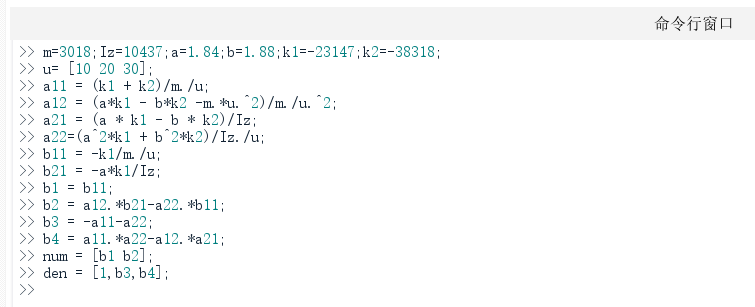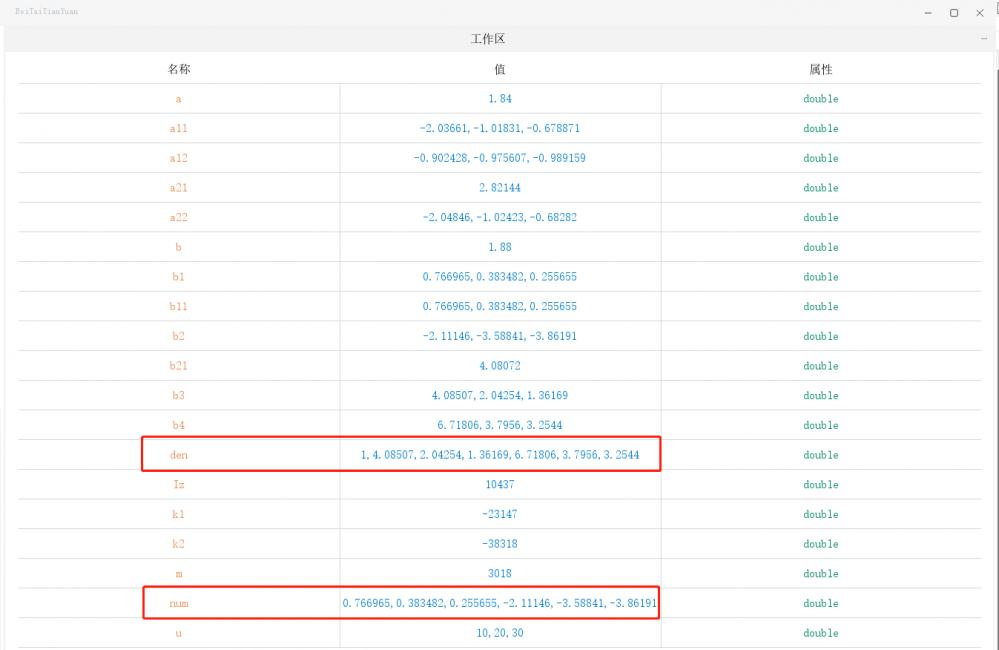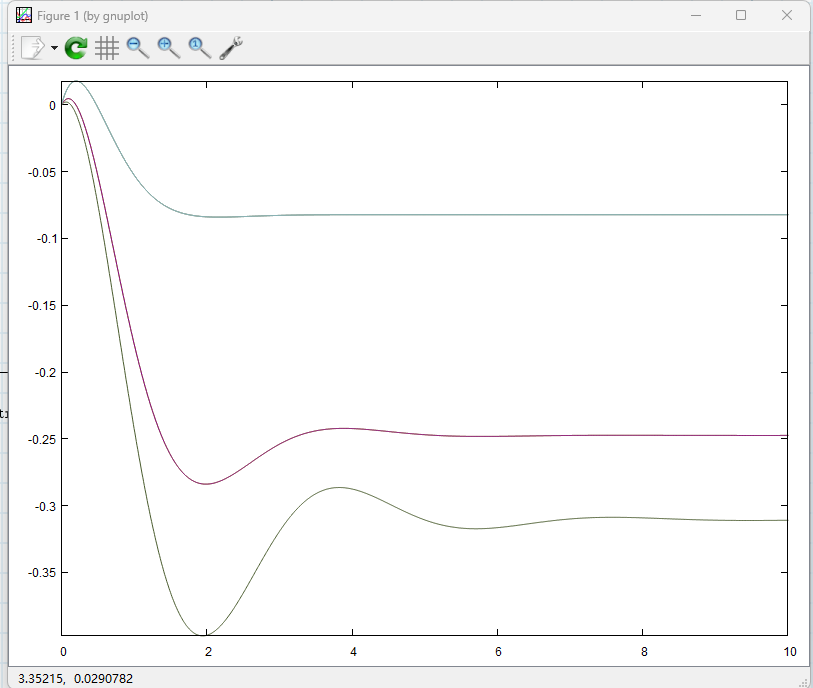0 2023-04-17

（1）假设 ω = 1, ζ = 0, 0.8, 1.5;

（2）假设 ζ = 1, ω = 1, 2, 3;

G(s1) = [1; 1 0 1];

G(s2) = [1; 1 1.6 1];

G(s3) = [1; 1 3 1];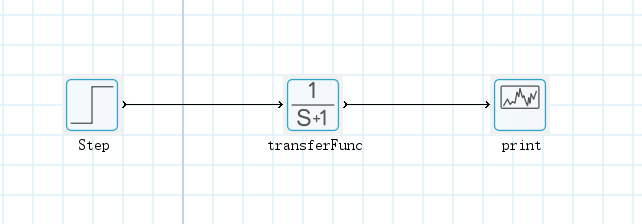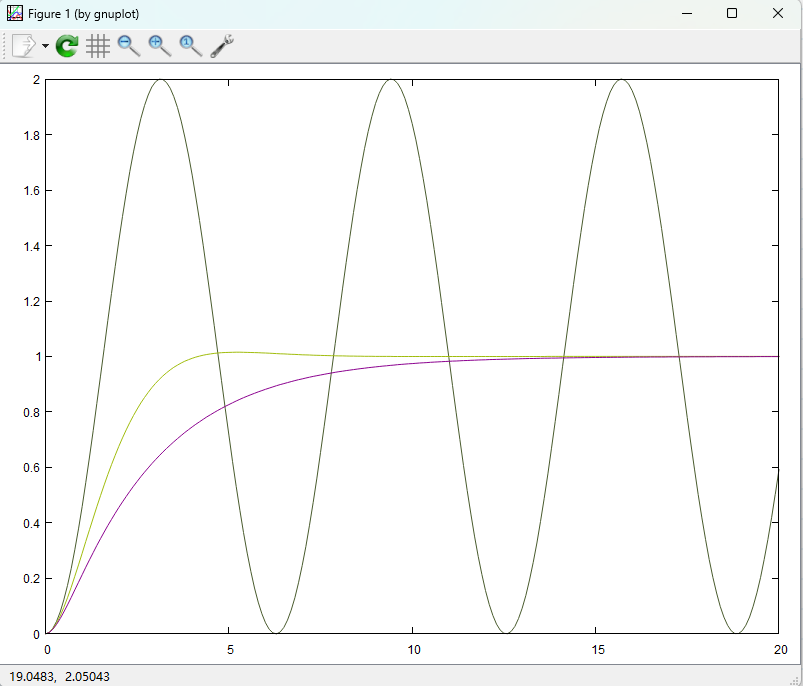G(s1) = [1; 1 0.5 1];

G(s2) = [4; 1 1 4];

G(s3) = [9; 1 1.5 9];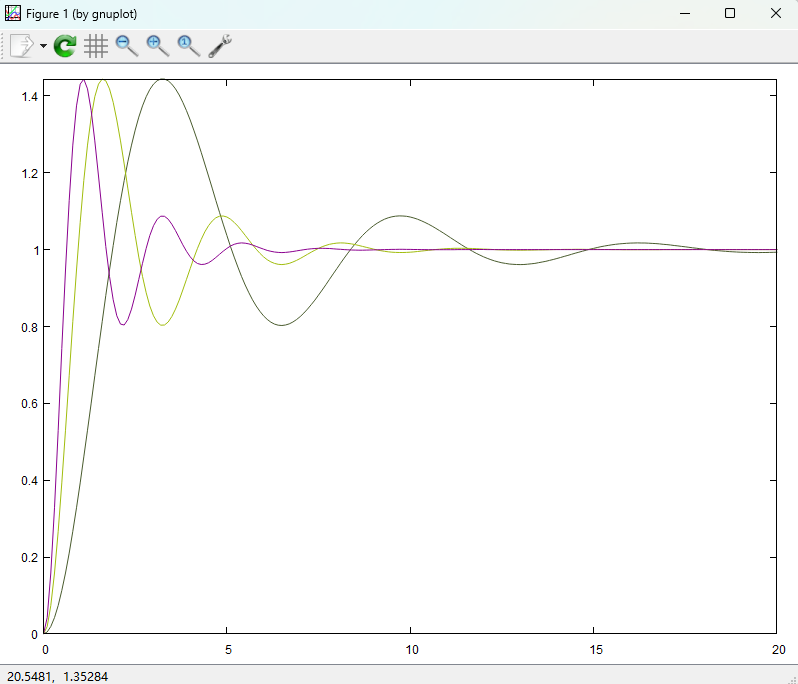0 2023-04-17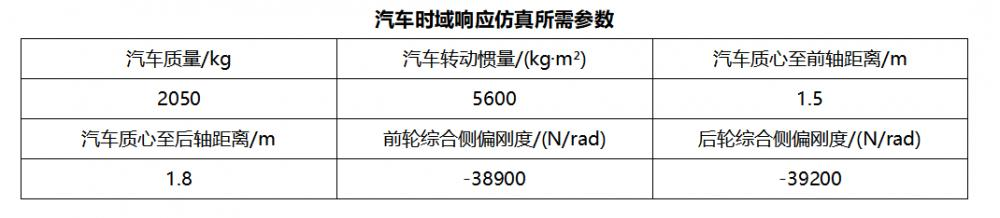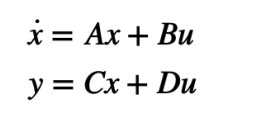>> m=2050;Iz=5600;a=1.5;b=1.8;L=3.3;

>> k1=-38900;k2=-39200;

>> u= [20 30 40];

>> a11 = (k1 + k2)/m./u;

>> a12 = (a*k1 - b*k2 -m.*u.^2)/m./u.^2;

>> a21 = (a*k1 - b*k2)/Iz;

>> a22=(a^2*k1 + b^2*k2)/Iz./u;

>> b11 = -k1/m./u;

>> b21 = -a*k1/Iz;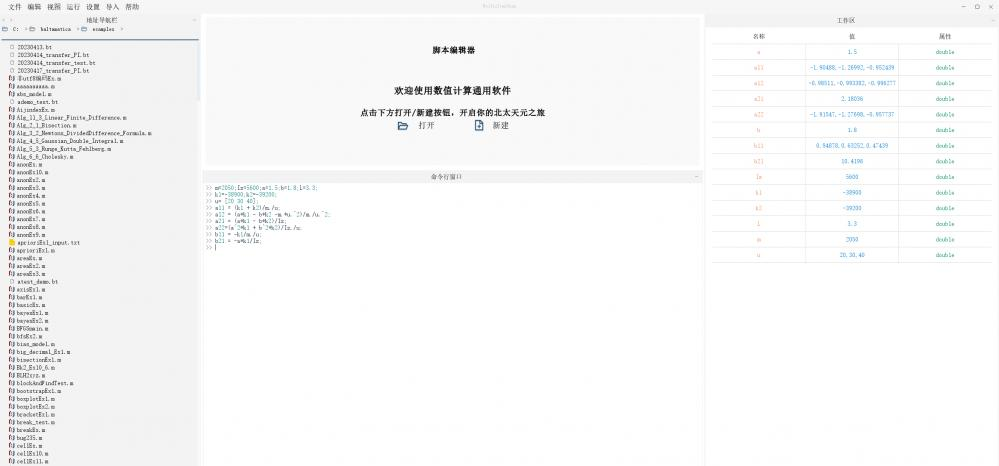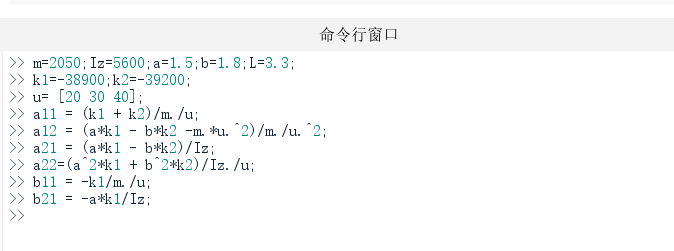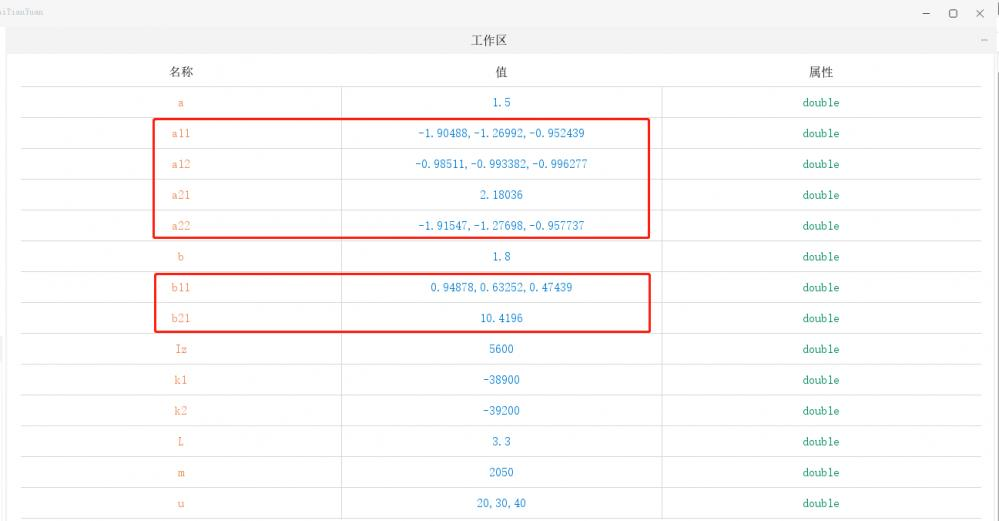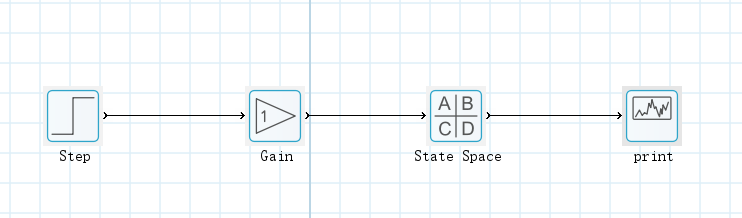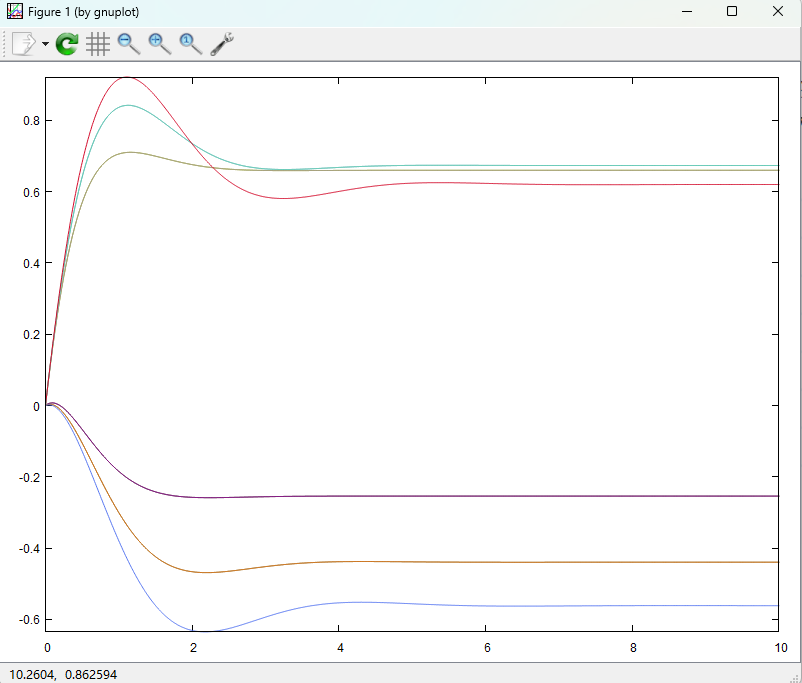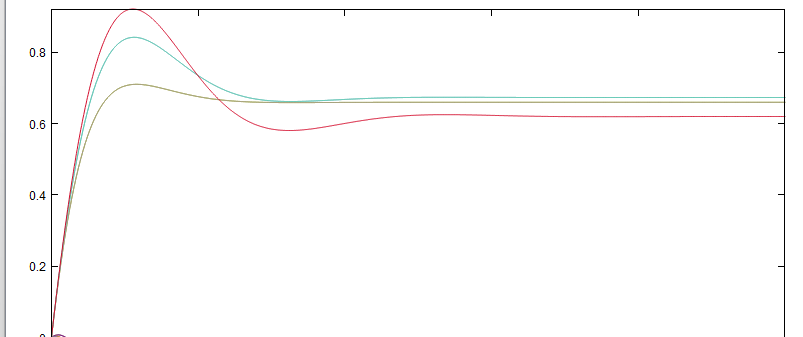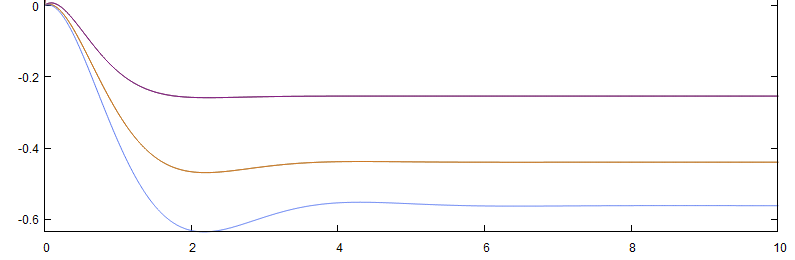0 2023-04-17

4:1衰减法控制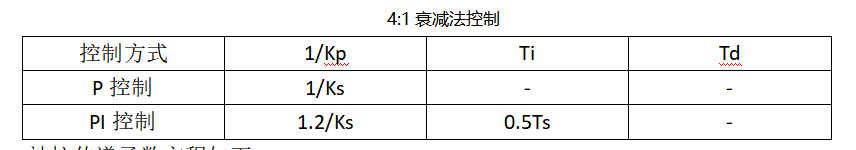Gp(s) =1 / 100^3 + 80^s + 17s + -1;

两个峰值之间的时间周期 Ts = 21.9967；

P控制：比例系数 Kp = Ks = 4.74；

PI控制：比例系数 Kp = Ks/1.2 = 3.95；

积分时间常数 Ti = 0.5 * Ts = 10.9984;

积分系数 Ki = Kp / Ti = 3.95 / 10.9984 = 0.3591;

P（比例）控制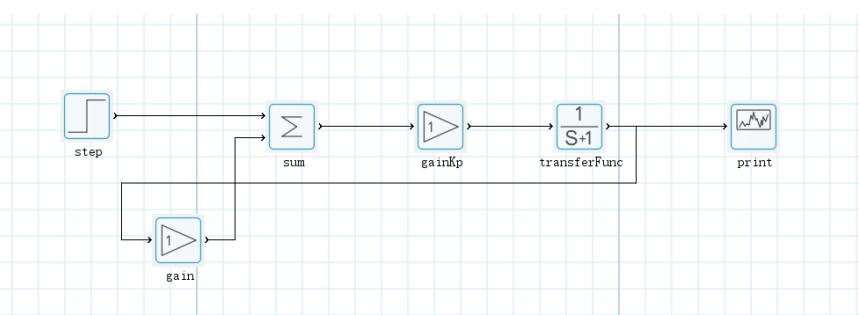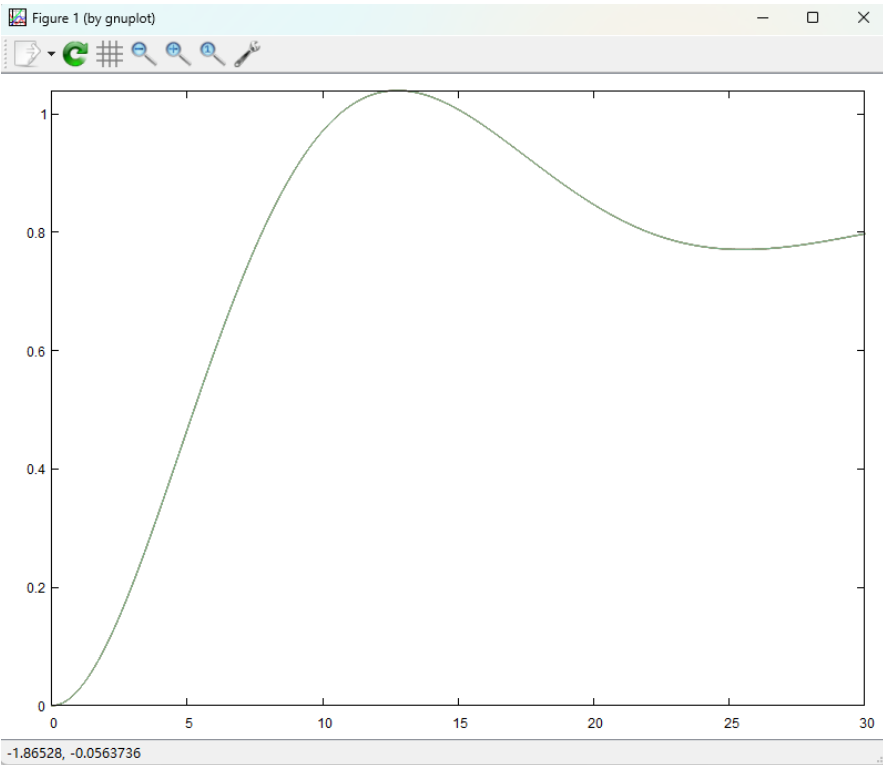PI（比例积分）控制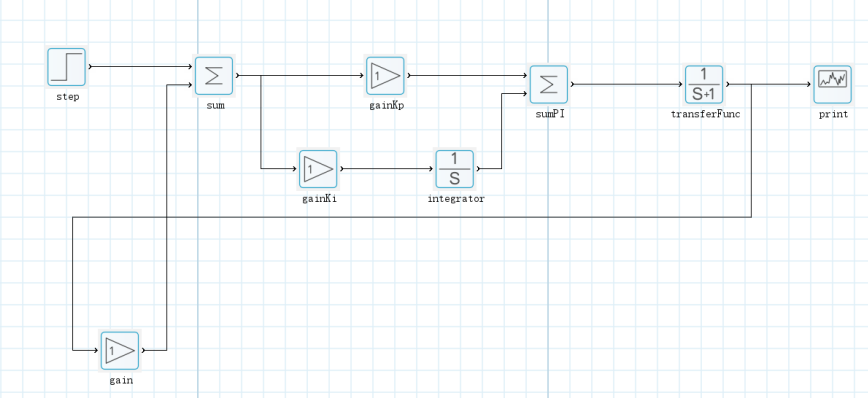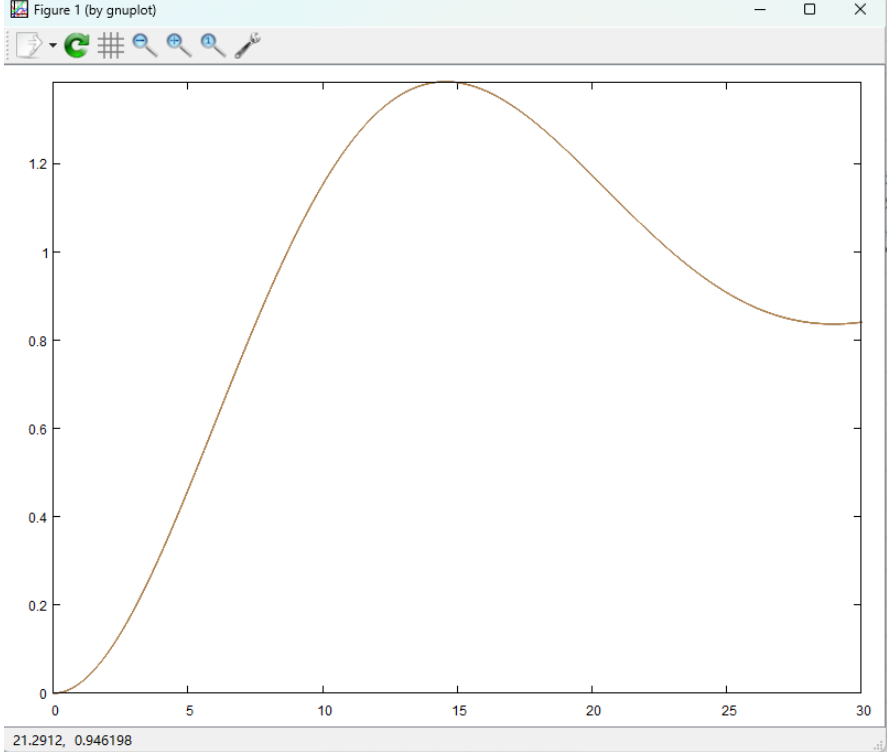0 2023-04-21